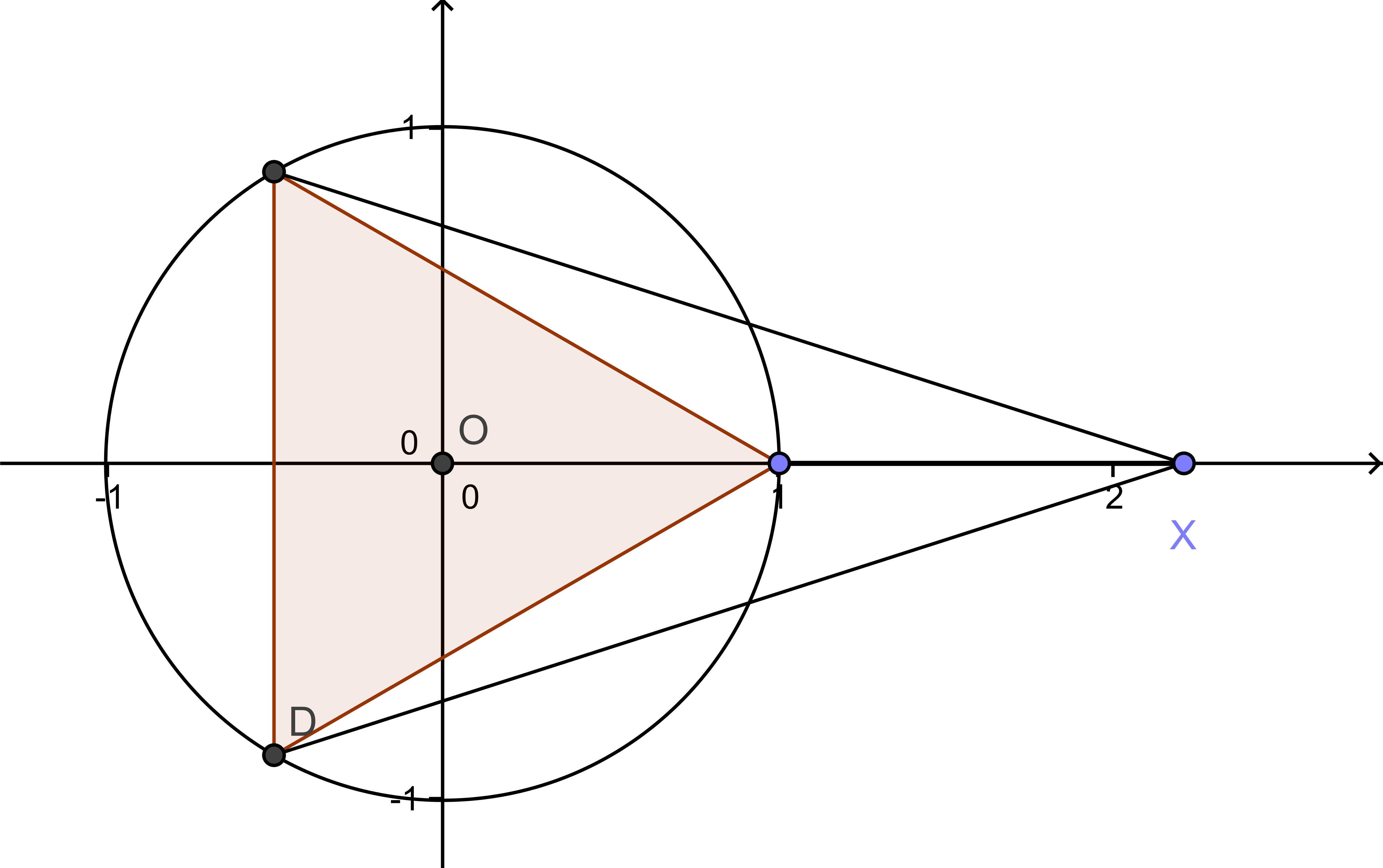# 上帝是一位算术家还是一位几何学家？（下）

──复数的引入对柏拉图主义的支持

$$\displaystyle s^3+t^3+3st(s+t)=3p(s+t)+2q$$

$$s=\sqrt{q-\sqrt{q^2-p^3}}, t=\sqrt{q+\sqrt{q^2-p^3}}$$

$$y=\sqrt{q-\sqrt{q^2-p^3}}+\sqrt{q+\sqrt{q^2-p^3}}$$。

$$\displaystyle y=\sqrt{\sqrt{-1}}+\sqrt{-\sqrt{-1}}$$.

$$\displaystyle s=\sqrt{-1},t=-\sqrt{-1}$$
$$\displaystyle s=-\frac{\sqrt{3}}{2}-\frac{\sqrt{-1}}{2},t=-\frac{\sqrt{3}}{2}+\frac{\sqrt{-1}}{2}$$
$$\displaystyle s=\frac{\sqrt{3}}{2}-\frac{\sqrt{-1}}{2},t=\frac{\sqrt{3}}{2}+\frac{\sqrt{-1}}{2}$$

$$p\sqrt{p}(4\cos^3\theta-3\cos\theta)=q$$，

$$\cos3\theta=\frac{q}{p\sqrt{p}}$$.

$x^2-1=(x-1)(x+1)$
$x^3-1=(x-1)(x^2+x+1)$
$x^4-1=(x-1)(x+1)(x^2+1)$
$x^5-1=(x-1)(x^2+\frac{1-\sqrt{5}}{2}x+1)(x^2+\frac{1+\sqrt{5}}{2}x+1)$

$x^3-1=(x-1)\sqrt{(x+\frac{1}{2})^2+(\frac{\sqrt{3}}{2})^2}\cdot\sqrt{(x+\frac{1}{2})^2+(-\frac{\sqrt{3}}{2})^2}$$$(x-x_i)(x-\bar{x_i})=\left | x-x_i \right |^2$$.

：这是《复分析，可视化方法》第一章中的两个不起眼的实例。在这本书中，你可以看到更多有关复数客观实在性的例子。